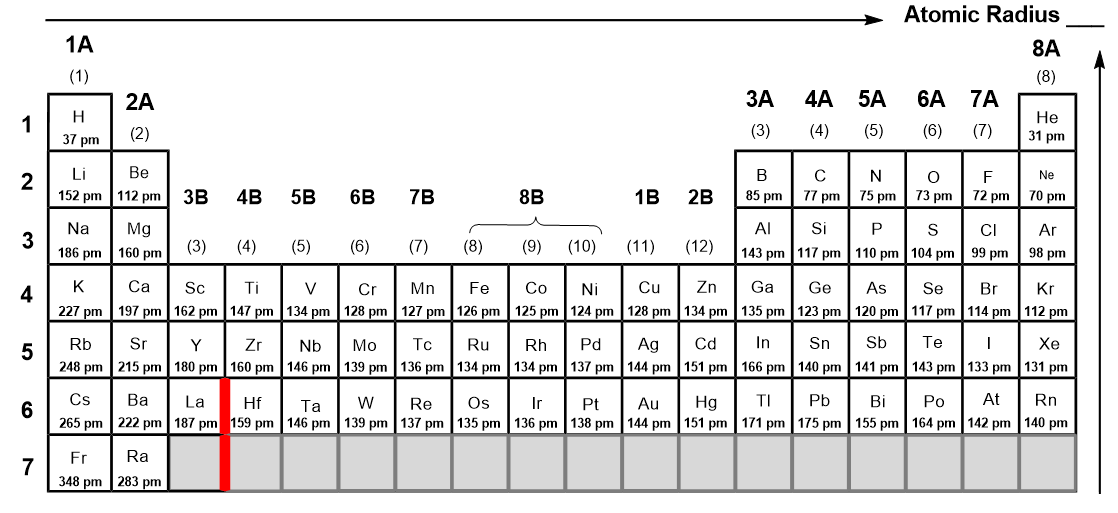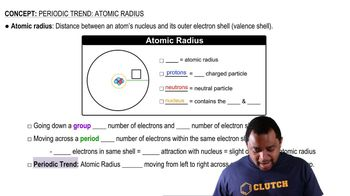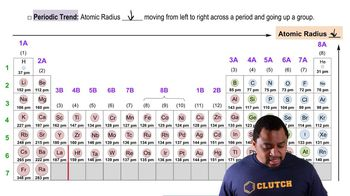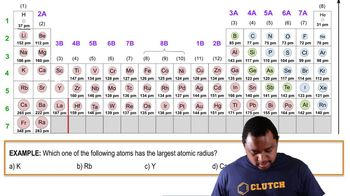Start typing, then use the up and down arrows to select an option from the list.
2:12 minutes
Problem 22
Textbook Question

# Which of the following statements about the bonding atomic radii in Figure 7.7 is incorrect? (i) For a given period, the radii of the representative elements generally decrease from left to right across a period. (ii) The radii of the representative elements for the n = 3 period are all larger than those of the corresponding elements in the n = 2 period. (iii) For most of the representative elements, the change in radius from the n = 2 to the n = 3 period is greater than the change in radius from n = 3 to n = 4. (iv) The radii of the transition elements generally increase moving from left to right within a period. (v) The large radii of the Group 1 elements are due to their relatively small effective nuclear charges.Verified Solution
This video solution was recommended by our tutors as helpful for the problem above.
232views

### Watch next

Master Periodic Trend: Atomic Radius with a bite sized video explanation from Jules Bruno

Start learning02:0201:4200:56